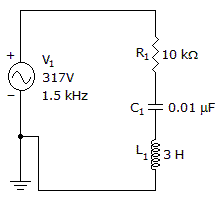# Electronics - RLC Circuits and Resonance

### Exercise :: RLC Circuits and Resonance - General Questions

46.

What is the voltage across R1, C1, and L1?A. VR = 156 V, VC = 165 V, VL = 441 V B. VR = 178 V, VC = 187 V, VL = 503 V C. VR = 219 V, VC = 232 V, VL = 619 V D. VR = 260 V, VC = 276 V, VL = 735 V

Answer: Option A

Explanation:

No answer description available for this question. Let us discuss.

#### Current Affairs 2021

Interview Questions and Answers